It is currently 20 Jun 2019, 11:05GMAT Club Daily Prep

Thank you for using the timer - this advanced tool can estimate your performance and suggest more practice questions. We have subscribed you to Daily Prep Questions via email.

Customized
for You

we will pick new questions that match your level based on your Timer History

Track

every week, we’ll send you an estimated GMAT score based on your performance

Practice
Pays

we will pick new questions that match your level based on your Timer History

Not interested in getting valuable practice questions and articles delivered to your email? No problem, unsubscribe here.If two points, A and B, are randomly placed on the circumferQuestion banks Downloads My Bookmarks Reviews Important topics
Author Message
TAGS:
GRE InstructorJoined: 10 Apr 2015
Posts: 1995
Followers: 60

Kudos [?]: 1811 , given: 9

If two points, A and B, are randomly placed on the circumfer [#permalink]
Expert's post00:00

Question Stats:50% (00:23) correct50% (00:56) wrongbased on 12 sessions
If two points, A and B, are randomly placed on the circumference of a circle with circumference 12pi inches, what is the probability that the length of chord AB is at least 6 inches?

(A) 1/(2pi)
(B) 1/pi
(C) 1/3
(D) 2/pi
(E) 2/3
[Reveal] Spoiler: OA

_________________

Brent Hanneson – Creator of greenlighttestprep.comGRE InstructorJoined: 10 Apr 2015
Posts: 1995
Followers: 60

Kudos [?]: 1811  , given: 9

Re: If two points, A and B, are randomly placed on the circumfer [#permalink]
3
KUDOS
Expert's post
GreenlightTestPrep wrote:
If two points, A and B, are randomly placed on the circumference of a circle with circumference 12pi inches, what is the probability that the length of chord AB is at least 6 inches?

(A) 1/(2pi)
(B) 1/pi
(C) 1/3
(D) 2/pi
(E) 2/3

Let's first determine the details of this circle.
For any circle, circumference = (diameter)(pi)

The circumference of the given circle is 12pi inches, so we can write: 12pi inches= (diameter)(pi)
This tells us that the diameter of the circle = 12 inches
It also tells us that the radius of the circle = 6 inches

Okay, now let's solve the question.
We'll begin by arbitrarily placing point A somewhere on the circumference.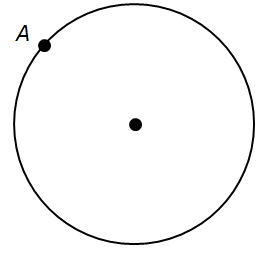So, we want to know the probability that a randomly-placed point B will yield a chord AB that is at least 6 inches long.
So, let's first find a location for point B that creates a chord that is EXACTLY 6 inches.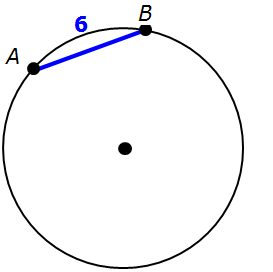There's also another location for point B that creates another chord that is EXACTLY 6 inches.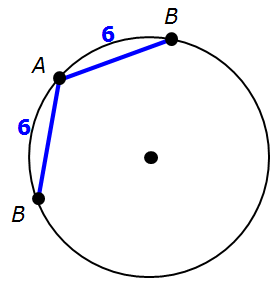IMPORTANT: For chord AB to be greater than or equal to 6 inches, point B must be placed somewhere along the red portion of the circle's circumference.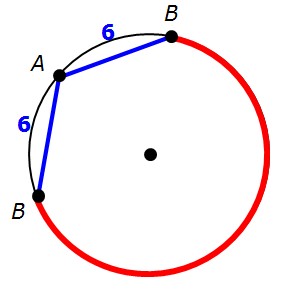So, the question really boils down to, "What is the probability that point B is randomly placed somewhere on the red line?"
To determine this probability, notice that the 6-inch chords are the same length as the circle's radius (6 inches)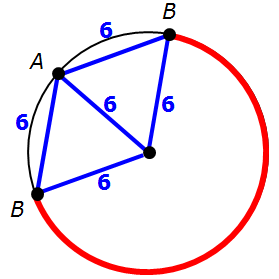Since these 2 triangles have sides of equal length, they are equilateral triangles, which means each interior angle is 60 degrees.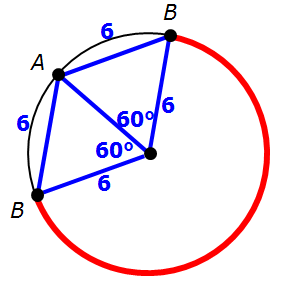The 2 central angles (from the equilateral triangles) add to 120 degrees.
This means the remaining central angle must be 240 degrees.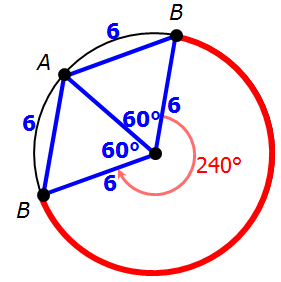This tells us that the red portion of the circle represents 240/360 of the entire circle.
So, P(point B is randomly placed somewhere on the red line) = 240/360 = 2/3

[Reveal] Spoiler:
E

_________________

Brent Hanneson – Creator of greenlighttestprep.comRe: If two points, A and B, are randomly placed on the circumfer   [#permalink] 29 May 2017, 16:42
Display posts from previous: Sort by

If two points, A and B, are randomly placed on the circumferQuestion banks Downloads My Bookmarks Reviews Important topicsPowered by phpBB © phpBB Group Kindly note that the GRE® test is a registered trademark of the Educational Testing Service®, and this site has neither been reviewed nor endorsed by ETS®.